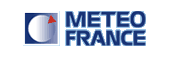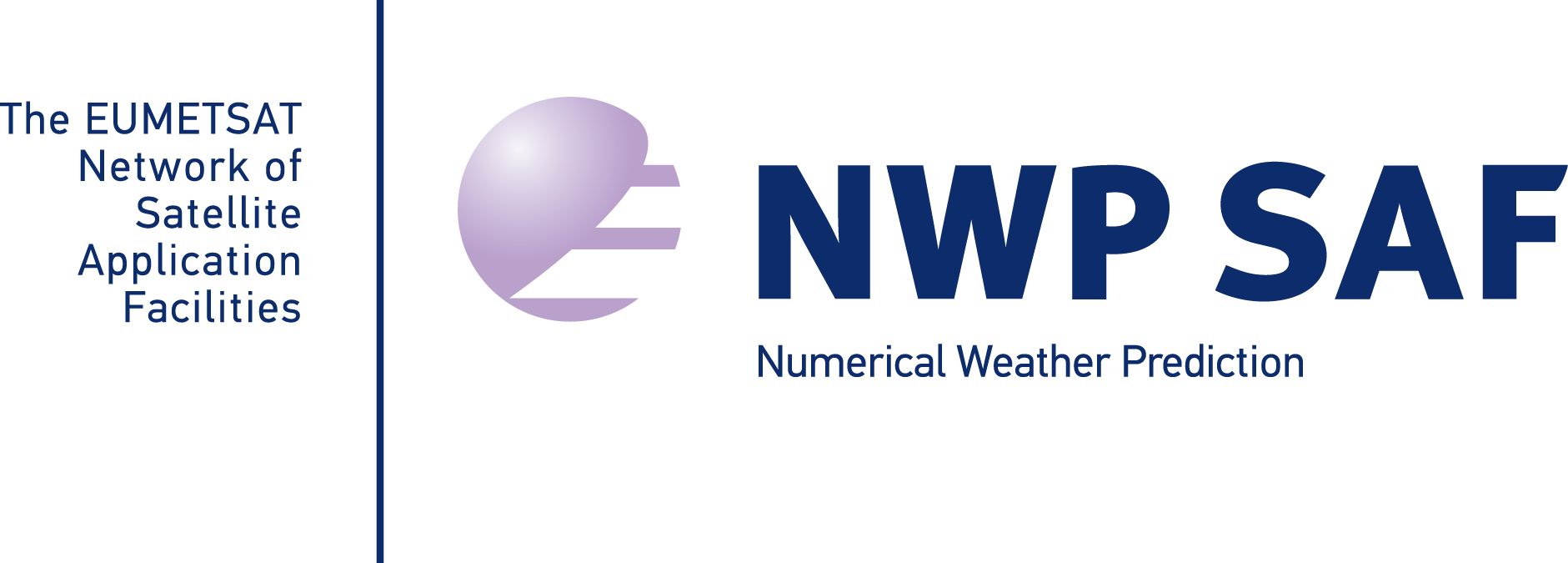Monthly monitoring statistics for each satellite scan position angles classes Radiances satellite observation typeEach graph shows curves radiances observations follow-up (brightness temperature) for each scan position angles and for each channel. These graphs are declined for each radiances sensor satellite and, for a given sensor, for each satellite and according to conditions of cloudiness (clear/cloudy) and/or the type of surface (sea/land/sea-ice). Statistics are computed on a monthly basis at the beginning of each month. On graphs are plotted : total number of observations (orange), of rejects (blue) and of gross errors (red) average and standard deviation curves of first-guess deviation after bias correction With a graph produced for a given sensor, satellite and channel we can examine evolution monitoring statistics parameters (total number of observations, rejects, gross errors and biases and standard deviation of first-guess deviation after bias correction) according to considered scan position angles classes.